# Metalens Design and Simulation with RSoft and CODE V

## Tools Used: CODE V, DiffractMOD, FullWAVE, BeamPROP

An effective simulation approach for metalens design is demonstrated by combining multiple simulation algorithms. Finite-Difference Time-Domain (FDTD) or Rigorous Coupled Wave Analysis (RCWA) is used to calculate the phase delay of various individual nano-cells, and then efficient beam propagation methods are used to trace the beam through the metalens or its equivalent phase mask. In this example we show that this multi-algorithm combined approach is both accurate and efficient, with significantly reduced demands on computational memory and time compared to a full FDTD metalens simulation.

## Introduction

A metalens is a difficult structure to design and simulate using photonic simulation algorithms, since its overall size is very large and contains many nano-structures. To simulate the nano-structures, a rigorous algorithm such as FDTD must be used. However, FDTD is computationally expensive, requiring substantial computer resources. The largest metalens that can be directly simulated in most labs through FDTD is only tens of micrometers in diameter, much smaller than what is needed .

In practice, however, direct FDTD simulation of the entire metalens is fortunately not required. In this paper, we demonstrate an approach that decomposes the metalens into simpler elements that can be solved individually. A rigorous algorithm such as FDTD, or alternatively RCWA, is used to calculate the phase shift of the individual unit nano-cells, from which a phase mask for the complete metalens is constructed.  More efficient algorithms, such as Beam Propagation Method (BPM) or Beam Synthesis Propagation (BSP), are then used to simulate the propagation through the metalens. For the propagation, we will use both BPM and BSP on the same metalens structures for cross-validation.

## Simulation Flow

The phase of each unit nano-cell is critical to metalens performance. Approximate estimation based on the phase shift of the mode guided in an isolated nano-cell is not accurate  because it ignores resonances inside the nano-cell and interference from neighboring nano-cells.  In our study, we use RSoft’s FullWAVE (FDTD) and DiffractMOD (RCWA) tools to calculate the phase delay for individual nano-cells with certain lattice patterns. Shown in Figure 1 are the calculated phase shifts as functions of normalized diameter for the nano-pillar and nano-hole (left), and for rectangular and hexagonal lattices of nano-pillars (right) at λ=532nm.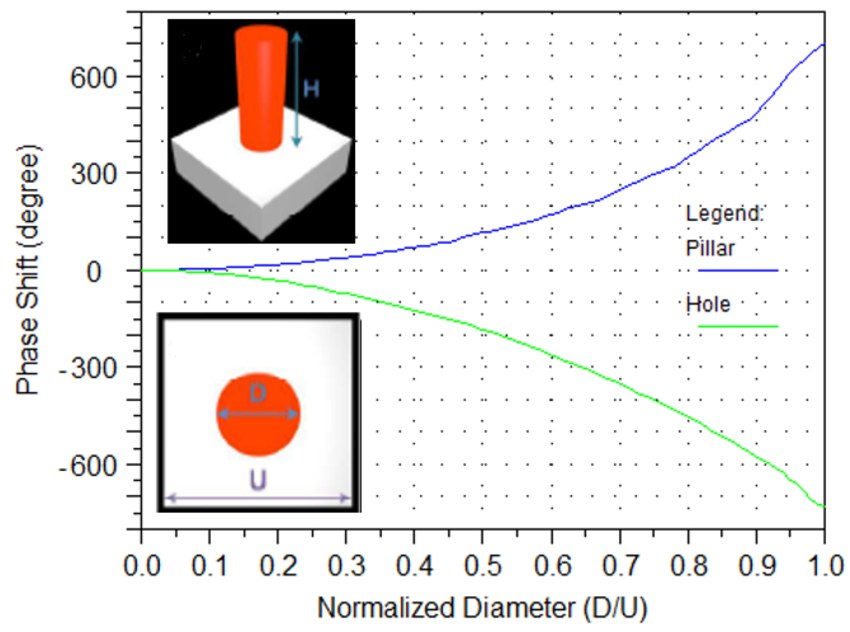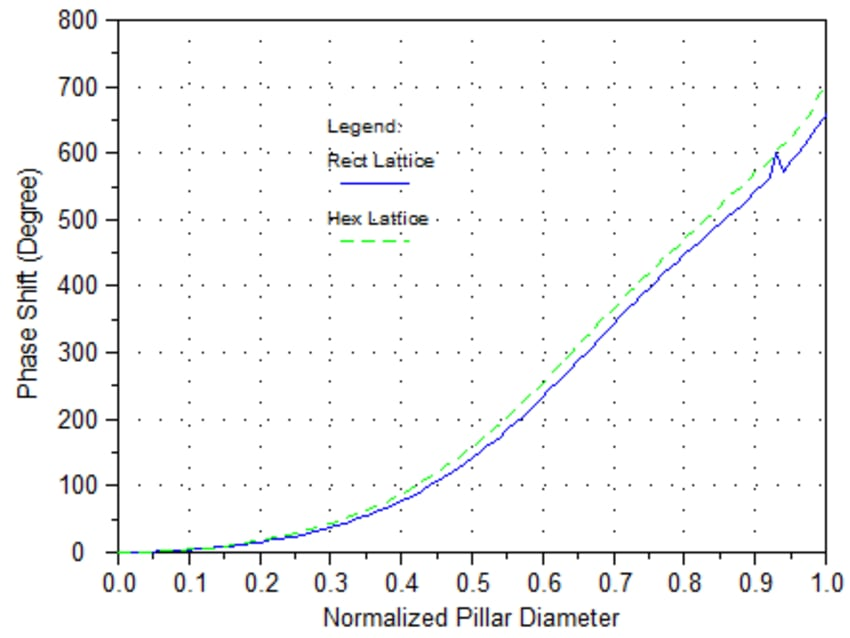Figure 1: Phase shift at λ=532nm of nano-pillar and nano-hole (left); for different lattices (right)

It is clearly seen that nano-pillars create a positive phase shift while nano-holes give a negative phase shift. It is also seen that the lattice pattern makes little difference; what matters is the normalized diameter that is associated with the fill factor.

## Layout of the metalens

Based on the phase delay curves shown in Figure 1, the diameter of each cell in a metalens can be determined according to the designed phase shift at that particular point. Shown in Figure 2 is the layout of an ideal metalens.

The phase profile of this metalens is shown in Equation 1.where λ is the wavelength and F is the focal length. The metalens is designed with a focal length of F=200µm, and a diameter of D=100µm, for green light (λ=532nm).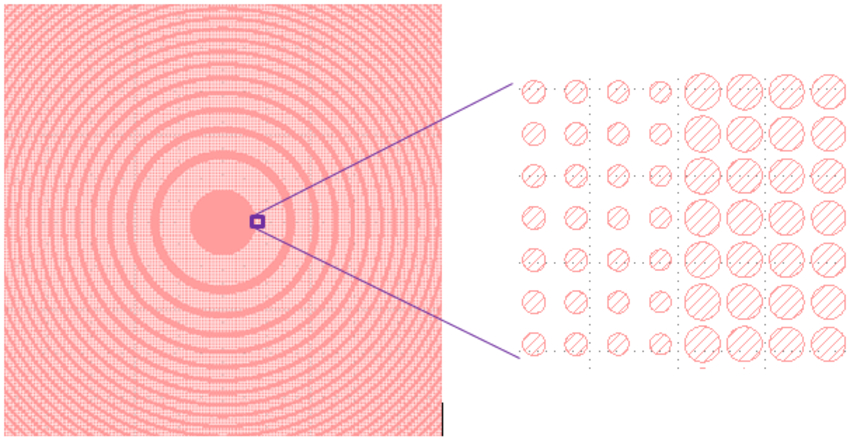Figure 2: Layout of an ideal metalens built from nano-pillars

To ensure that the metalens we create is manufacturable, the normalized diameters are chosen in the range of [0.45,0.85], which can provide 360o phase shift. The phase mask of the above metalens is generated and is shown in Figure 3.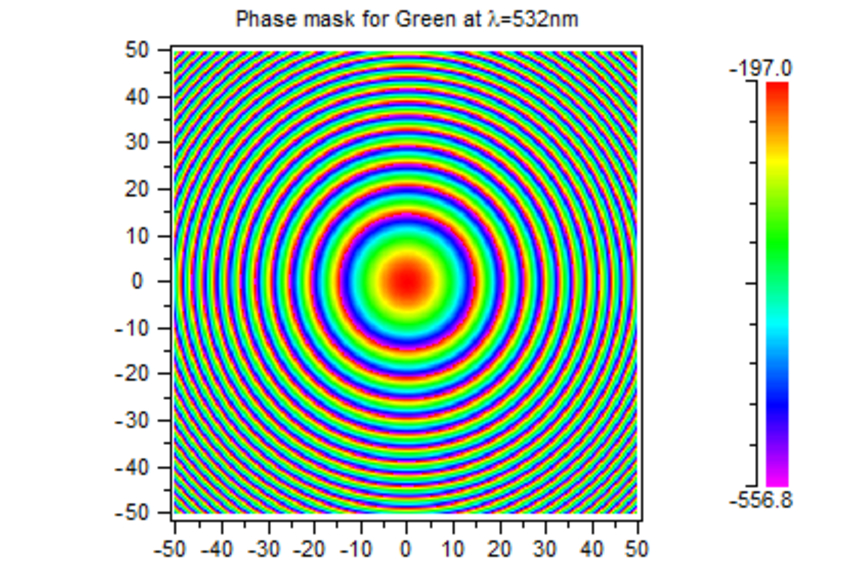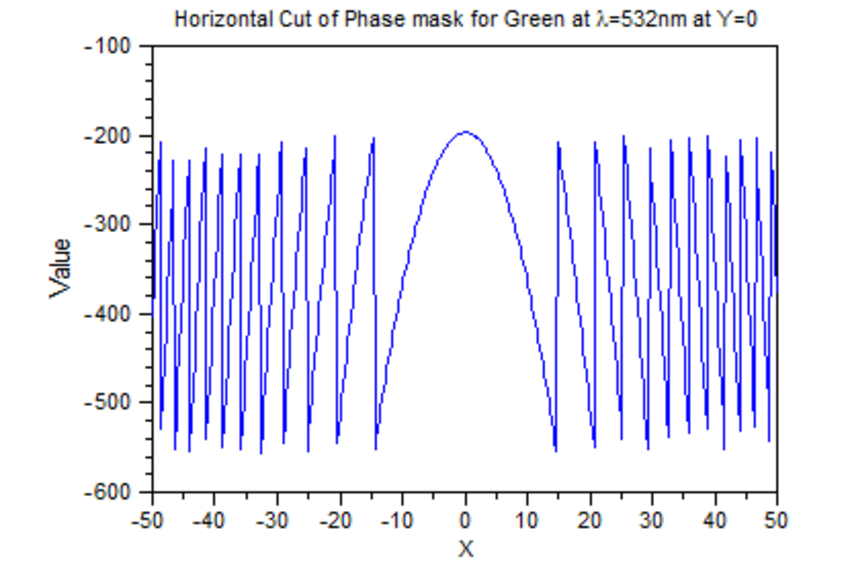Figure 3: Phase mask of the metalens

## Validation of BPM algorithm on a small structure

BPM is an efficient method to simulate forward propagation without accounting for backward reflections. We first validate BPM against the bidirectional FDTD algorithm on a small metalens 20µm in diameter and NA=0.25. The theoretical focal length is F=17.3µm. Shown in Figure 4 on the left is the BPM simulation result with F=16.96µm, and on the right is the FDTD result with F=17.14µm. This comparison shows that BPM agrees very well with FDTD for this application.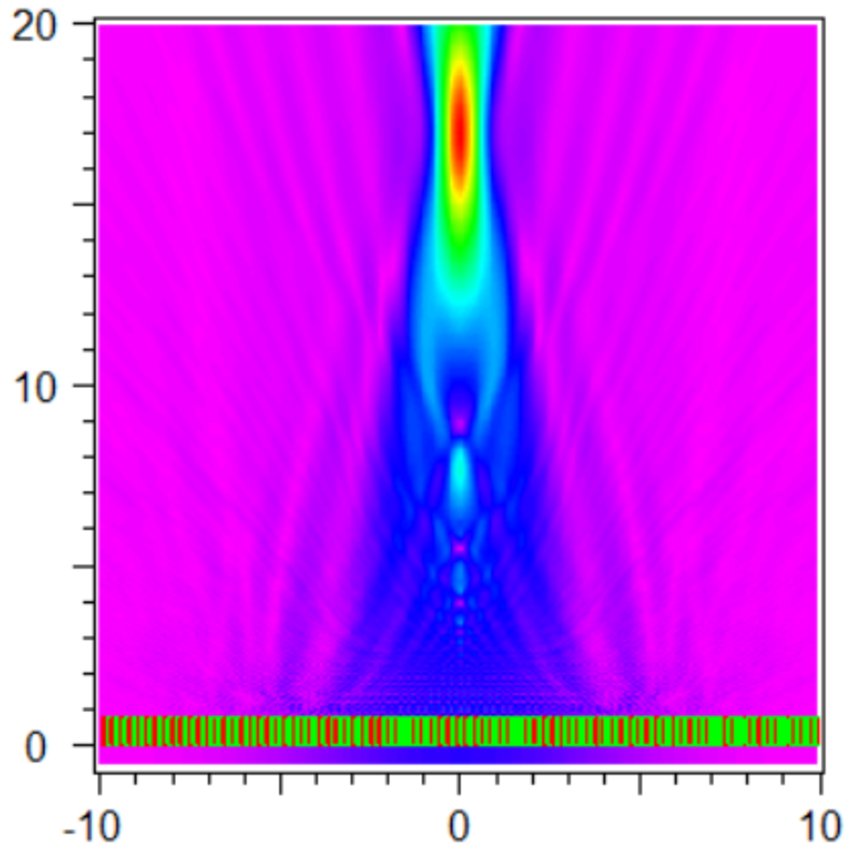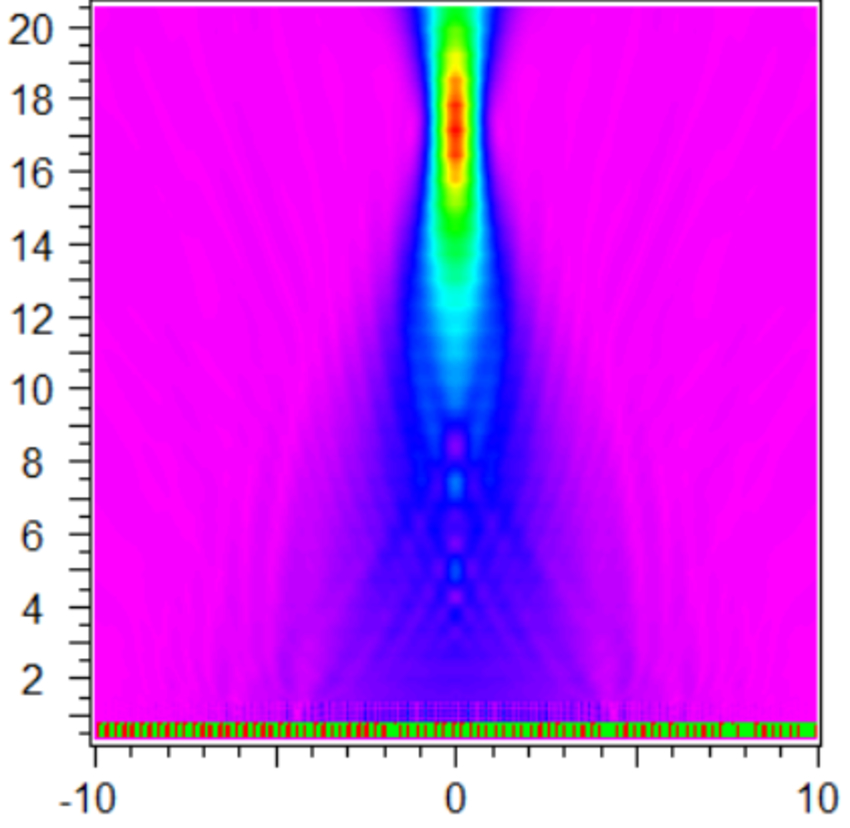Figure 4: BPM result (left) and FDTD result (right)

For comparison the memory requirements for BPM were 0.19G and FDTD was 55G, and the respective simulation times on a desktop computer were 1.5mins and  130 minutes. Thus, BPM provides similar results to FDTD in significantly faster simulation time.

## Validation of BPM algorithm on a larger scale structure

A larger test structure is shown in Figure 2 with diameter =100µm and F=200µm.  Shown in Figure 5 on the left is the BPM result, using a Gaussian field as the launch excitation. The calculated focal length is F=200.1µm. The memory and time required were ~3.3GB RAM and 4 minutes on a desktop computer.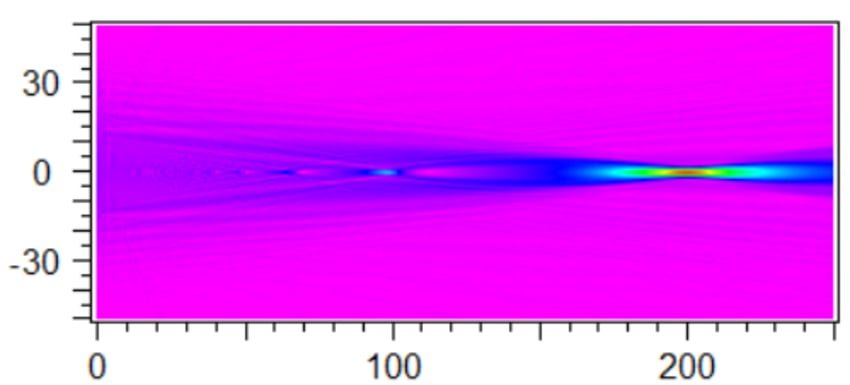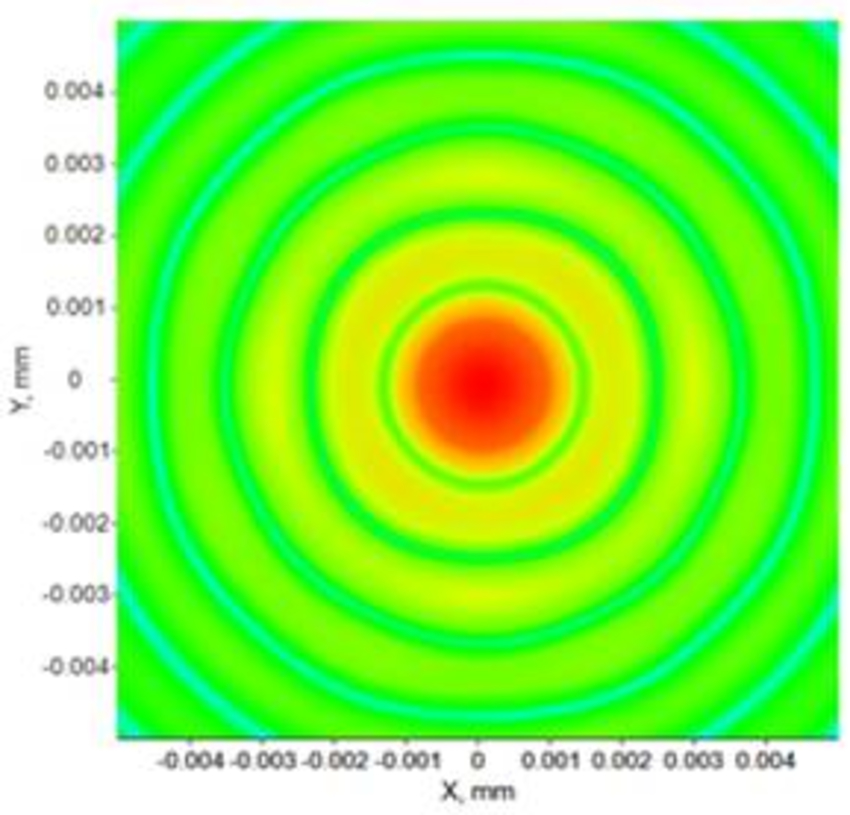Figure 5: BPM result (left) and BSP result (right) for green light (λ=532nm) at L=200µm

Because of the huge memory requirements that would be required for FDTD to simulate the large metalens directly, we validate BPM against another propagation algorithm, Synopsys’ CODE V® Beam Synthesis Propagation (BSP) feature. BSP, due to its implementation, does not handle the physical optics simulation of the nano-structures directly.  Instead, it treats the metalens as a phase mask, as shown in Figure 3. The BSP result is shown in Figure 5 on the right. It confirms the smallest spot size obtained occurs at L=200µm, in excellent agreement with the BPM result. For this simulation, the BSP simulation takes approximately 3 minutes with 4 GB RAM on a laptop computer.

## Dispersion of the metalens

Chromatic dispersion of the metalens is a well-known problem. Accurate modeling of the dispersion is the key step to design of an achromatic metalens. As shown in Figure 6, the nano-pillar under study behaves very differently for each RGB wavelength, even having some phase jumps for blue and green light caused by resonances in the nano-pillar.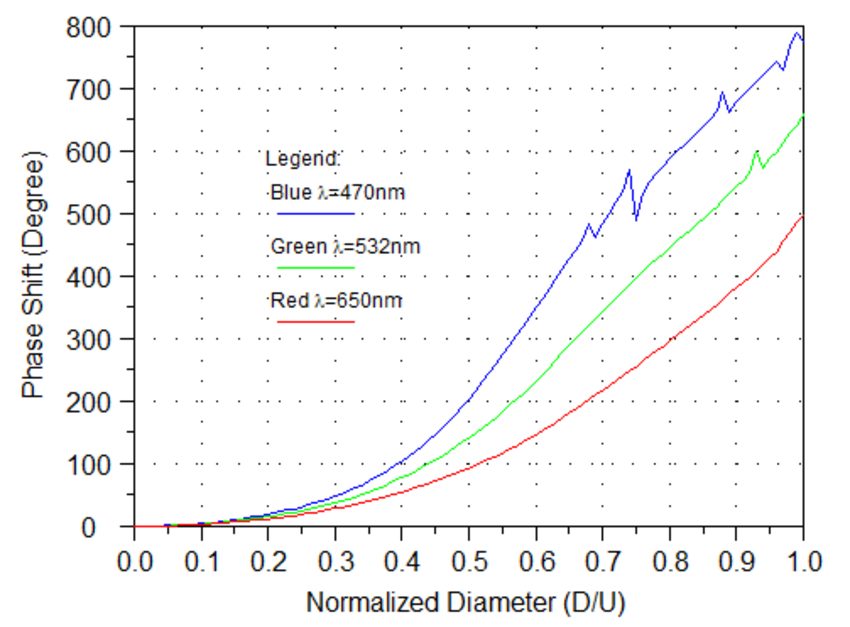Figure 6: Dispersion of the nano-pillar

Based on the above curves, we can generate phase masks for blue and red light. These generated phase masks and BSP propagations are used to predict the metalens focal lengths as 223um and 161nm for blue and red light respectively. For comparison, we also run direct BPM simulations of the metalenses; the focal lengths predicted via BPM are 223 µm and 161 µm, in agreement with the BSP results.  The RMS fit of the beam profile was approximately λ/100 in each case.

The BPM propagations and transverse field profiles at the foci are shown in Figure 7.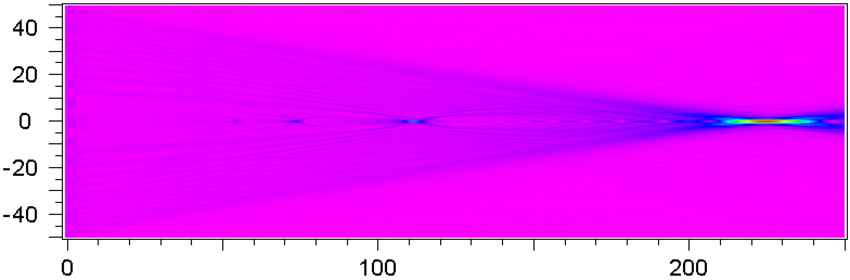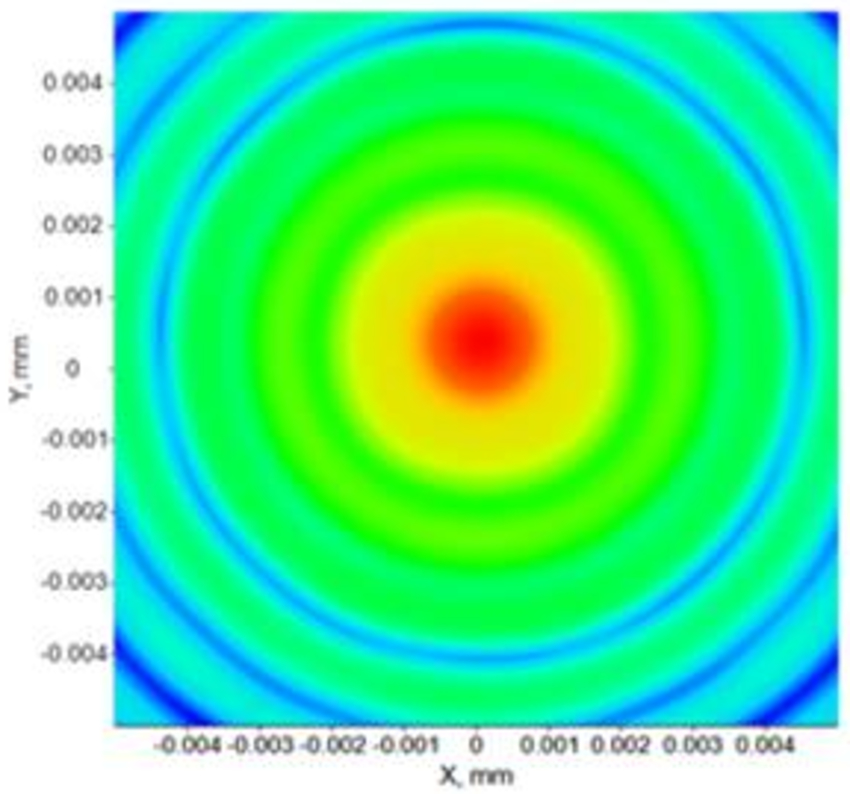Figure 7: BPM result (left) and BSP result (right) for blue light (λ=470nm) at L=220µm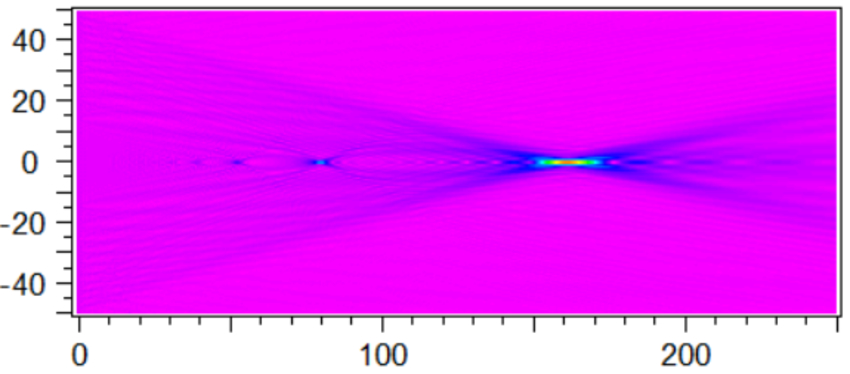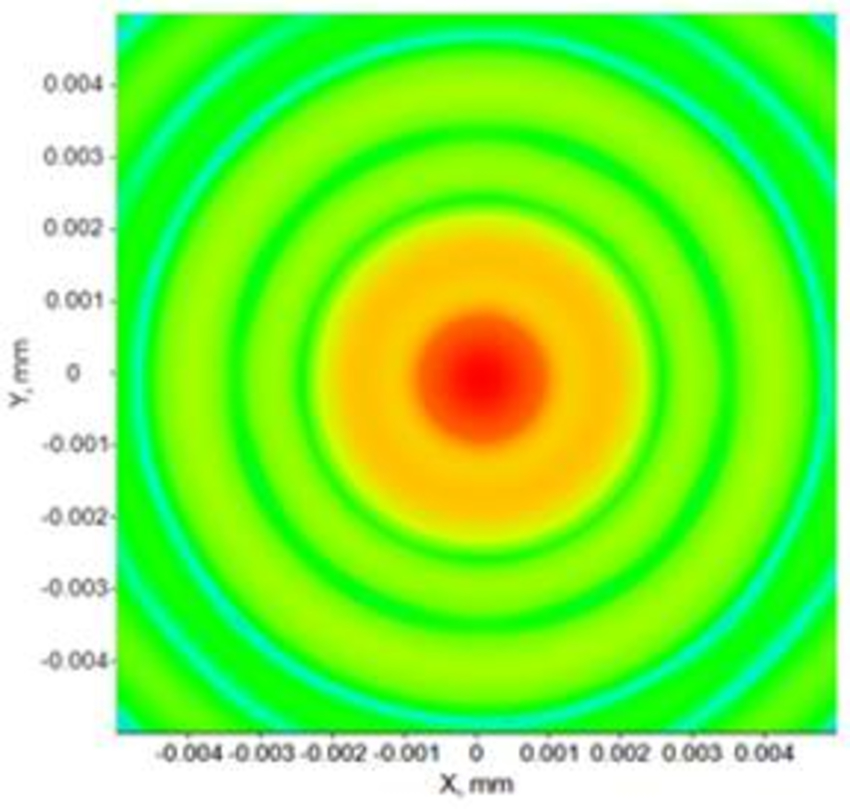Figure 8: BPM result (left) and BSP result (right) for red light (λ=650nm) at L=160µm

## Conclusions

We have demonstrated an effective approach to designing and simulating practical metalenses by using RSoft’s FullWAVE and/or DiffractMOD to simulate the individual metalens nano-cells and BeamPROP or CODE V's BSP features to simulate the propagation of the light through the whole metalens. The test examples shown here demonstrate that this is a viable approach, and provides both accuracy and efficient use of computational resources.

## References

    Khorasaninejad, Mohammadreza, et al. "Polarization-insensitive metalenses at visible wavelengths." Nano letters 16.11 (2016): 7229-7234.